# How to Get Color of the Cell Using VBA in Microsoft Excel 2010

In this article, you will learn how to get color of the cell using VBA code.

We need to follow the below steps to launch VB editor.

Click on Developer tab

From Code group, select Visual BasicClick on Insert, and then ModuleThis will create a new module.

Enter the following code in the Module

Function ColorIndex(CellColor As Range)

ColorIndex = CellColor.Interior.ColorIndex

End Function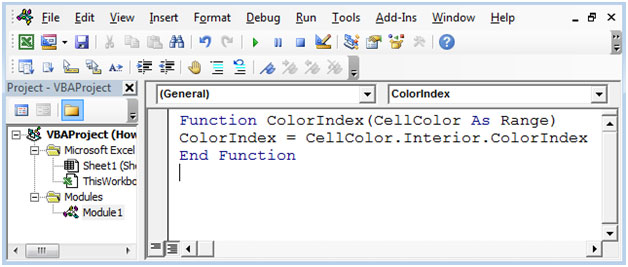To get the color of the below cells, refer below snapshot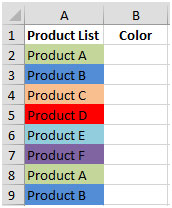In cell B2, enter the formula as =ColorIndex(A2) & then copy down the formula in below cells.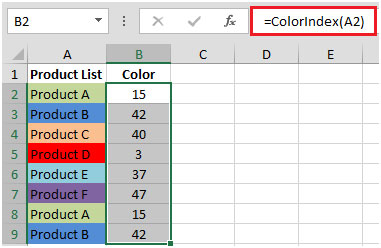Let us take one more example:

To know how many times a particular color has repeated (count by color), refer below snapshot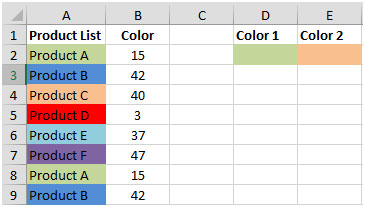We can use COUNTIF function along with newly created UDFColorIndex function.

COUNTIF: Counts the number of cells within a range that meets the condition.

Syntax: =COUNTIF(range,criteria)

range: It refers to the range of selected cells from which the criteria will check the number of items that have found.

criteria: The criteria define which cells to count.

In cell D2, the formula would be =COUNTIF(\$B\$2:\$B\$9,ColorIndex(D2))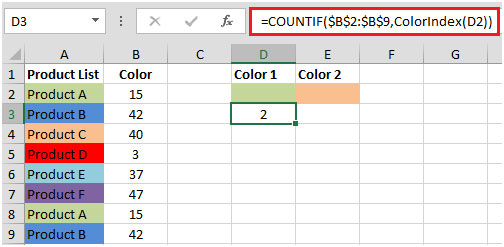By copying the formula in cell E2, we will get the count by colors.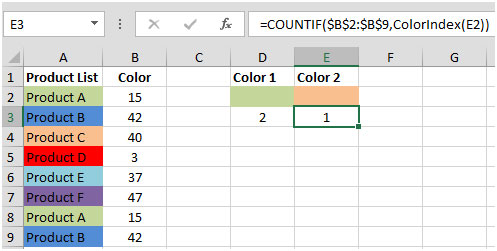This is how we can get the color of any cell.

1.Thank you, this is great.

Is there any way to make the formula volatile (update as soon as the cell value changes)?

•Yes, you can use VBA sheet_change event. Here is the link.

2.Thank you very much!

3.Hi ,

My issues is like , If cell A2 color is Green , i want to replicate the same color in another cell A8.. not color value.

for example.
A2 cell color = green , then A8 color is also green.
if i change A2 color into red = A8 also turn into red.

Kinldy help into this.

••